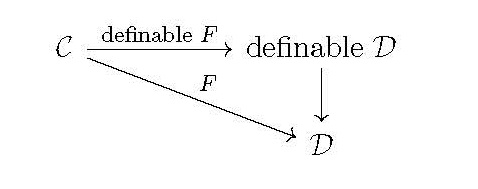## Oliver Club

Jeffrey BergfalkKurt Gödel Research Center, University of Vienna
Better invariants
Friday, November 12, 2021 - 4:00pm
Malott 532 (Lounge)

Abstract: Many of the classical functors $F:\mathcal{C}\to\mathcal{D}$ of homological algebra and algebraic topology factor through a definable version'' of the category $\mathcal{D}$, as in the following diagram:The corresponding definable $F$'' provides significantly stronger invariants for the spaces or groups in $\mathcal{C}$, particularly when the latter are limit objects such as the solenoid (pictured). As examples, we describe definable Ext and $\mathrm{lim}^1$ and \v{C}ech cohomology functors, showing that each distinguishes among uncountable classes of objects whose corresponding classical invariants are constant. As the terminology definable'' might suggest, this work represents an infusion of descriptive set theoretic techniques into algebraic contexts; it is joint with Martino Lupini and Aristotelis Panagiotopoulos.

Refreshments will be served at 3:30 PM.